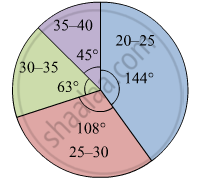# The Age Group and Number of Persons, Who Donated Blood in a Blood Donation Camp is Given Below. Draw a Pie Diagram from It. - Algebra

Diagram
The age group and number of persons, who donated blood in a blood donation camp is given below. Draw a pie diagram from it.
 Age group(Yrs) 20 - 25 25 - 30 30 - 35 35 -40 No. of persons 80 60 35 25

#### Solution

The measures of central angles are given  in the table.

 Age group(Yrs) No. of persons Central Angle 20 - 25 80 $\frac{80}{200} \times 360 = 144^\circ$ 25 - 30 60 $\frac{60}{200} \times 360 = 108^\circ$ 30 - 35 35 $\frac{35}{200} \times 360 = 63^\circ$ 35 - 40 25 $\frac{25}{200} \times 360 = 45^\circ$ Total 200

The pie digram showing the above data is given below:Concept: Concept of Pie Graph (Or a Circle-graph)
Is there an error in this question or solution?

#### APPEARS IN

Balbharati Mathematics 1 Algebra 10th Standard SSC Maharashtra State Board
Chapter 6 Statistics
Practice Set 6.6 | Q 1 | Page 163